## Online Education for RS Aggarwal Class 9 Solutions Chapter 13 Volume and Surface Area Ex 13A

These Solutions are part of RS Aggarwal Solutions Class 9. Here we have given RS Aggarwal Class 9 Solutions Chapter 13 Volume and Surface Area Ex 13A.

Other Exercises

Question 1.
Solution:
(i) Length of cuboid (l) = 12cm
and height (h) = 4.5cm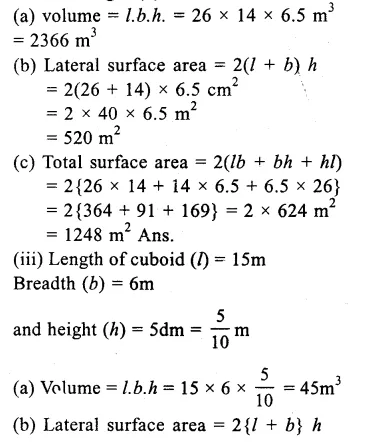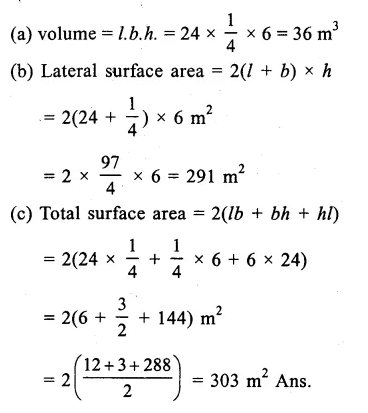Question 2.
Solution:
Length of closed rectangular cistern (l) = 8m
and depth (b) = 2.5m.
(i) .’. Volume of cistern = l.b.h.
= 8 x 6 x 2.5 m³ = 120m³
(ii) Total surface area = 2(lb + bh + hl)
= 2(8 x 6 + 6 x 2.5 + 2.5 x 8) cm²
= 2(48 + 15 + 20)
= 2 x 83 m²
= 166 m² Ans.

Question 3.
Solution:
Length of room (l) = 9m
and height (h) = 6.5m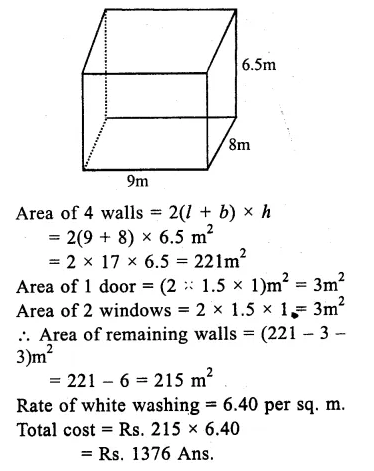Question 4.
Solution:
Length of pit (l) = 20m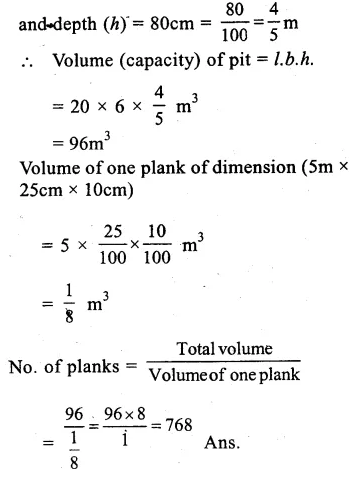Question 5.
Solution:
Length of wall (l) = 8m.
Width (b) = 22.5 cm = $$\frac { 225 }{ 10X100 } =\frac { 9 }{ 40 } m$$
and height (h) = 6m.
Volume of wall = l.b.h.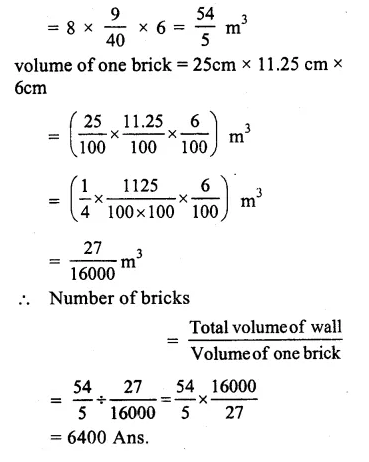Question 6.
Solution:
Length of wall (l) = 15m.
Width (b) = 30cm = $$\frac { 30 }{ 100 } =\frac { 3 }{ 10 } m$$
Height (h) = 4m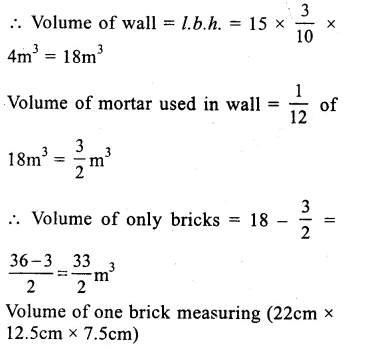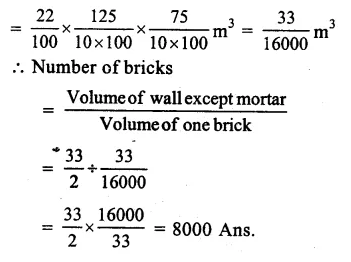Question 7.
Solution:
Outer length of opened cistern = 1.35m = 135 cm
Breadth = 1.08 m = 108 cm
Depth = 90cm
Thickness of iron = 2.5cm.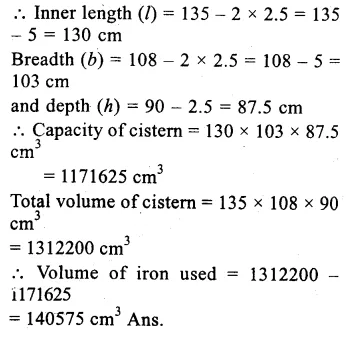Question 8.
Solution:
Depth of river = 2m
width = 45m.
Length of current in 60 minutes = 3km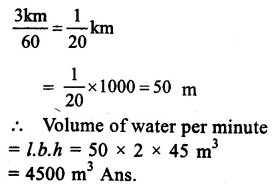Question 9.
Solution:
Total cost of box = Rs. 1620
Rate per sq. m = Rs. 30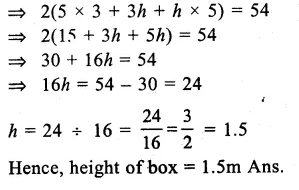Question 10.
Solution:
Length of room (l) = 10m
Height (h) = 5m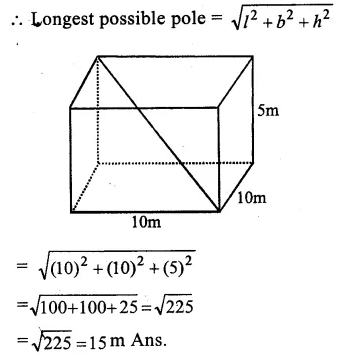Question 11.
Solution:
Length of hall (l) = 20m
and height (h) = 4.5m.
Volume of the air inside the hallQuestion 12.
Solution:
Length of class room (l) = 10m
Width (b) = 6.4 m
Height (h) = 5m.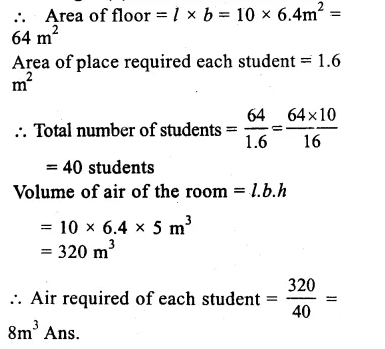Question 13.
Solution:
Volume of cuboid = 1536 m³
Length (l) = 16m
Ratio in breadth and height = 3:2
their height (h) = 2xQuestion 14.
Solution:
Length of cuboid (l) = 14 cm
Breadth (b) = 11 cm .
Let height (h) =x cm
Surface area = 2(lb + bh + hl)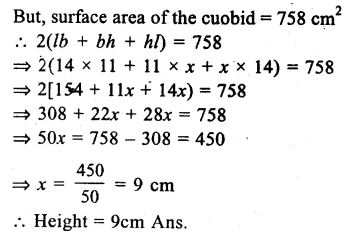Question 15.
Solution:
(a) Edge of cube (a) = 9m .
(i) volume = a³ = (9)³ m³ = 729 m³
(ii) Lateral surface area = 4a²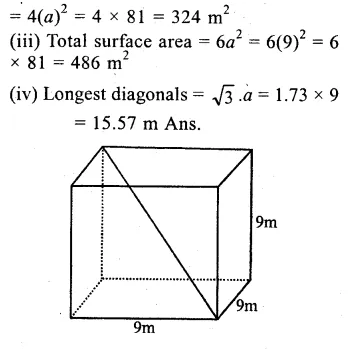Question 16.
Solution:
Total surface area of a cube = 1176 cm²
Let each edge he ‘a’
then 6a² =1176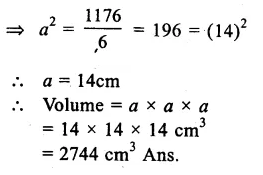Question 17.
Solution:
Lateral surface area of a cube = 900 cm²
Let ‘a’ be the edge of the cube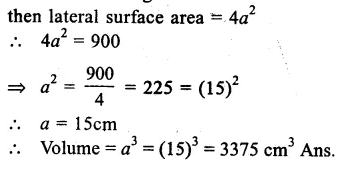Question 18.
Solution:
Volume of a cube = 512 cm³
Let ‘a’ be its edge, then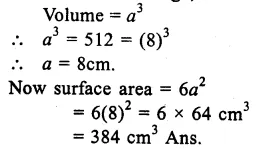Question 19.
Solution:
Edge of first-cube = 3 cm.
Volume = (3)³ = 27 cm³Question 20.
Solution:
Area of ground = 2 hectares
= 2 x 10000 = 20000 m²
Height of rain falls 5cm = $$\frac { 5 }{ 100 }$$m
∴ Volume of rain water = 20000 x $$\frac { 5 }{ 100 }$$ m³
= 1000 m³ Ans.

Hope given RS Aggarwal Class 9 Solutions Chapter 13 Volume and Surface Area Ex 13A are helpful to complete your math homework.

If you have any doubts, please comment below. Learn Insta try to provide online math tutoring for you.